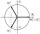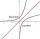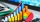Im>0?

Is -10i a positive number?

Result

Solution:

No, complex (imaginary) numbers are not ordered and therefore does not make sense to compare them.

Leave us a comment of this math problem and its solution (i.e. if it is still somewhat unclear...):Be the first to comment!To solve this verbal math problem are needed these knowledge from mathematics:

Try our complex numbers calculator.

Next similar math problems:

1. Imaginary numbersFind two imaginary numbers whose sum is a real number. How are the two imaginary numbers related? What is its sum?
2. Complex number coordinatesWhich coordinates show the location of -2+3i
3. Linear imaginary equationGiven that ? "this is z star" Find the value of the complex number z.
4. De Moivre's formulaThere are two distinct complex numbers z such that z3 is equal to 1 and z is not equal 1. Calculate the sum of these two numbers.
5. ReciprocalCalculate reciprocal of z=0.8-1.8i:
6. LogCalculate value of expression log |3 +7i +5i2| .
7. Is complexAre these numbers 2i, 4i, 2i + 1, 8i, 2i + 3, 4 + 7i, 8i, 8i + 4, 5i, 6i, 3i complex?
8. Theorem proveWe want to prove the sentence: If the natural number n is divisible by six, then n is divisible by three. From what assumption we started?
9. Fish tankA fish tank at a pet store has 8 zebra fish. In how many different ways can George choose 2 zebra fish to buy?
10. MedianThe number of missed hours was recorded in 11 pupils: 5,12,6,8,10,7,5,110,2,5,6. Determine the median.
11. Sequence 3Write the first 5 members of an arithmetic sequence: a4=-35, a11=-105.
12. SeatsSeats in the sport hall are organized so that each subsequent row has five more seats. First has 10 seats. How many seats are: a) in the eighth row b) in the eighteenth row
13. Six termsFind the first six terms of the sequence a1 = -3, an = 2 * an-1
14. 75th percentile (quartille Q3)Find 75th percentile for 30,42,42,46,46,46,50,50,54
15. EquationEquation ? has one root x1 = 8. Determine the coefficient b and the second root x2.
16. RootsDetermine the quadratic equation absolute coefficient q, that the equation has a real double root and the root x calculate: ?
17. Linsys2Solve two equations with two unknowns: 400x+120y=147.2 350x+200y=144